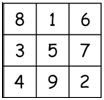# Magic Square

The magic square is a square matrix, whose order is odd and where the sum of the elements for each row or each column or each diagonal is same.The sum of each row or each column or each diagonal can be found using this formula. n(n2+ 1)/2

Here are the rules to construct a magic square −

• We will start from the middle column of the first row, of the matrix, and always go to the top left corner to place next number
• If the row exceeds, or the row is not in the matrix, then, change the column as left column and place the number at last row of the matrix, and go for top left corner again.
• If the column exceeds, or the column is not in the matrix, then change the row as top row and place the number at last column of that matrix, then go to the top left corner again.
• When the top left corner is not vacant or both row and column exceeds the range, then place the number at the bottom of the last-placed number.

## Input and Output

Input:
The order of the matrix 5
Output:
15 8  1  24 17
16 14 7  5  23
22 20 13 6   4
3  21 19 12 10
9  2  25 18 11

## Algorithm

createSquare(mat, r, c)

Input: The matrix.

Output: Row and Column.

Begin
count := 1
fill all elements in mat to 0
range := r * c
i := 0
j := c/2
mat[i, j] := count //center of top row

while count < range, do
increase count by 1
if both i and j crosses the matrix range, then
increase i by 1
else if only i crosses the matrix range, then
i := c – 1
decrease j by 1
else if only j crosses the matrix range, then
j := c – 1
decrease i by 1
else if i and j are in the matrix and element in (i, j) ≠ 0, then
increase i by 1
else
decrease i and j by 1
mat[i, j] := count
done
display the matrix mat
End

## Example

#include<iostream>
#include<iomanip>
using namespace std;

void createSquare(int **array, int r, int c) {
int i, j, count = 1, range;
for(i = 0; i<r; i++)
for(j = 0; j<c; j++)
array[i][j] = 0;    //initialize all elements with 0

range = r*c;
i = 0;
j = c/2;
array[i][j] = count;

while(count < range) {
count++;
if((i-1) < 0 && (j-1) < 0)    //when both row and column crosses the range
i++;
else if((i-1) <0) {    //when only row crosses range, set i to last row, and decrease j
i = r-1;
j--;
}else if((j-1) < 0) {    //when only col crosses range, set j to last column, and decrease i
j = c-1;
i--;
}else if(array[i-1][j-1] != 0)    //when diagonal element is not empty, go to next row
i++;
else{
i--;
j--;
}
array[i][j] = count;
}

// Printing the square
for(i = 0; i<r; i++) {
for(j = 0; j<c; j++)
cout <<setw(3) << array[i][j];
cout << endl;
}
}

main() {
int** matrix;
int row, col;
cout << "Enter the order(odd) of square matrix :";
cin >> row;
col = row;

matrix = new int*[row];

for(int i = 0; i<row; i++) {
matrix[i] = new int[col];
}
createSquare(matrix, row, col);
}

## Output

Enter the order(odd) of square matrix :5
15  8  1 24 17
16 14  7  5 23
22 20 13  6  4
3 21 19 12 10
9  2 25 18 11